Algebra 1 7-8 Guided Practice: Geometric Sequences
starstarstarstarstarstarstarstarstarstar
by Matthew Richardson
| 10 Questions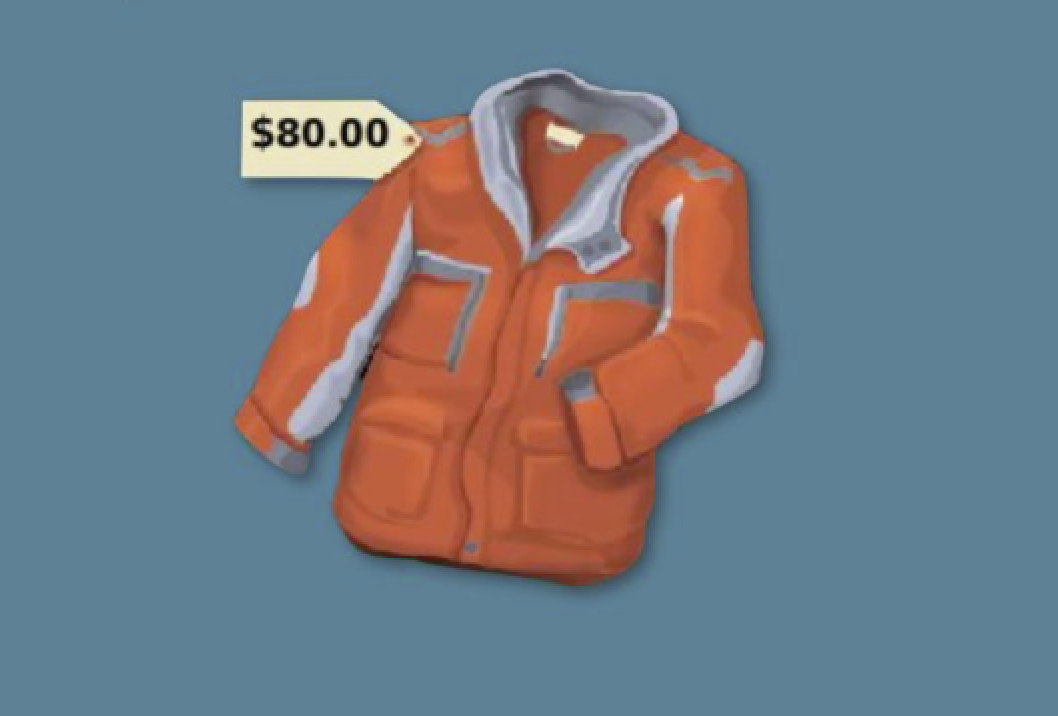1
1
10
Solve It! Imagine working part time at a clothing store. Each week a coat doesn't sell, its price is marked down 20%. What will the sale price for the coat be after three weeks of not selling? Enter the dollar amount in this form: \$71.23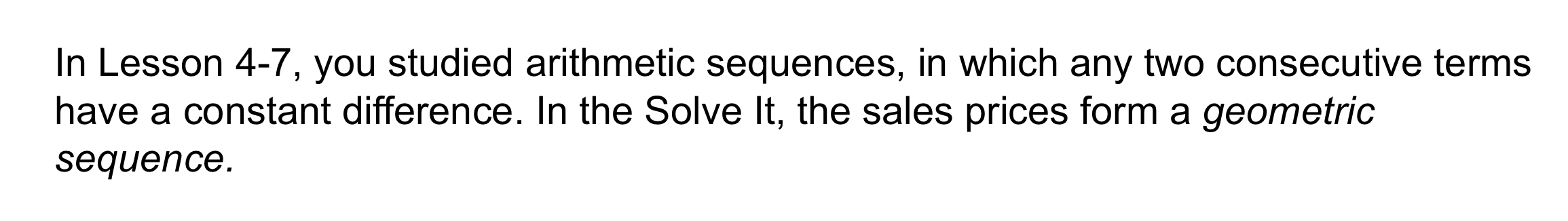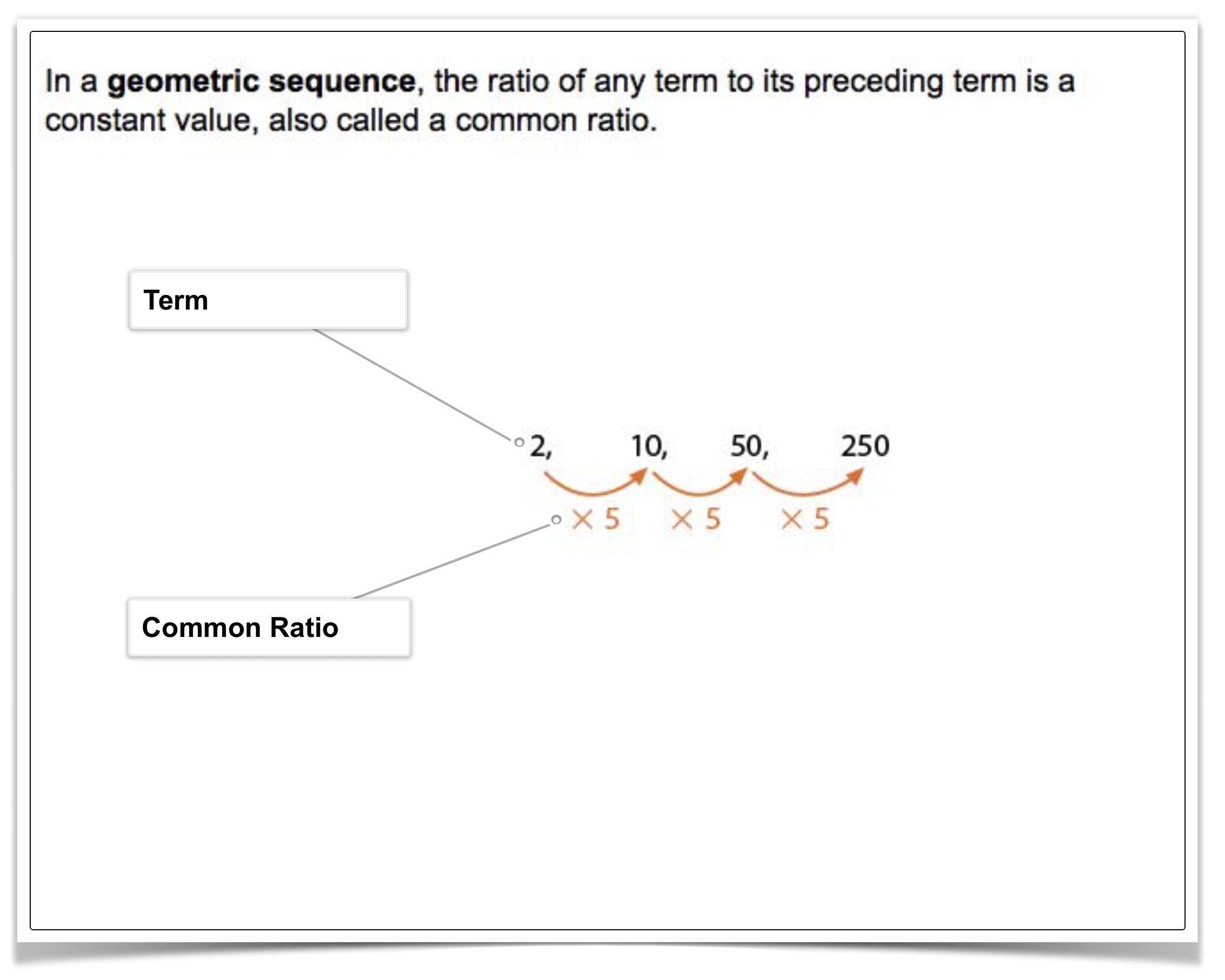2
2
10
Problem 1 Got It?
A
B
C
D3
3
10
Problem 1 Got It?
A
B
C
D4
4
10
Problem 1 Got It?
A
B
C
D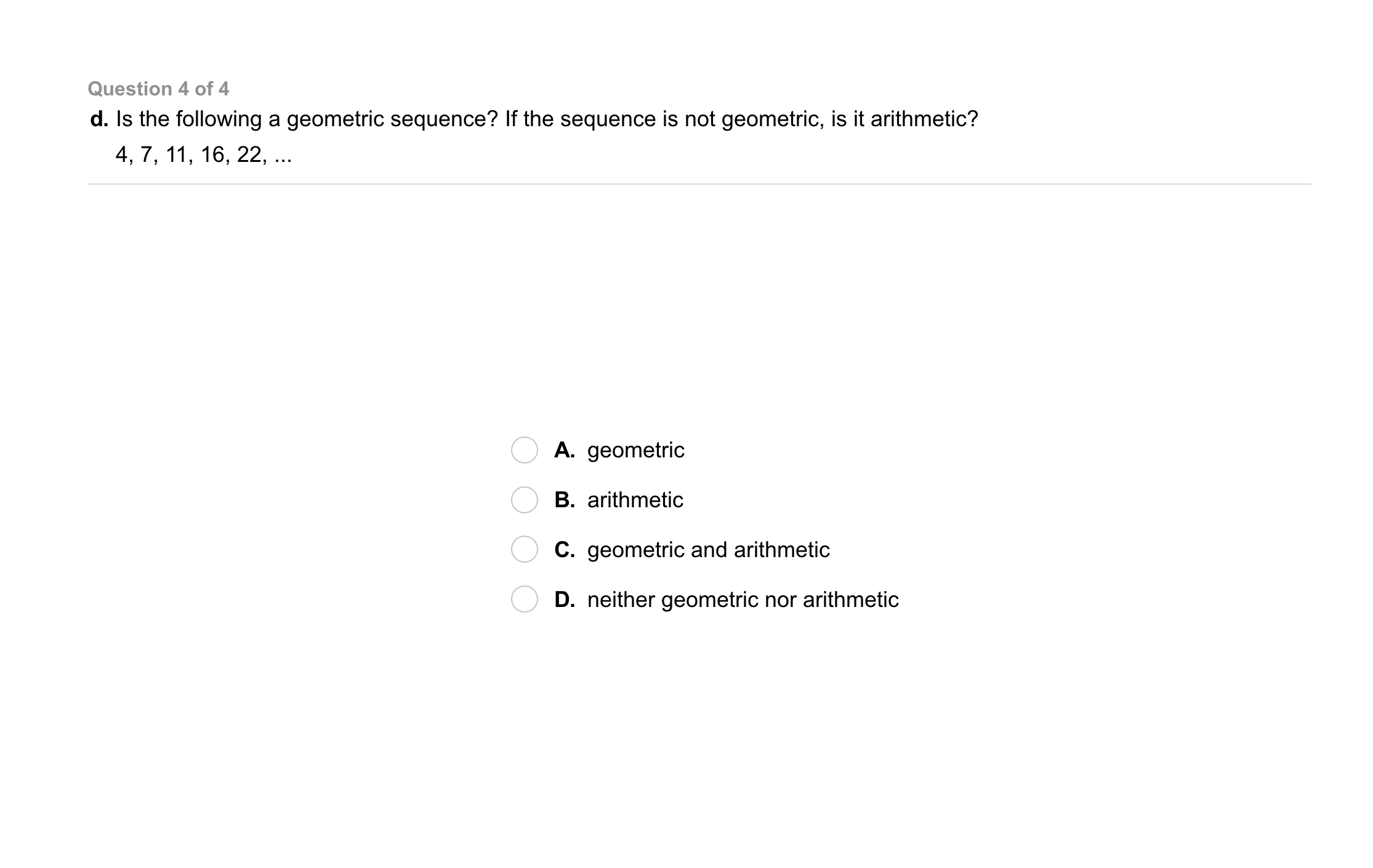5
5
10
Problem 1 Got It?
A
B
C
D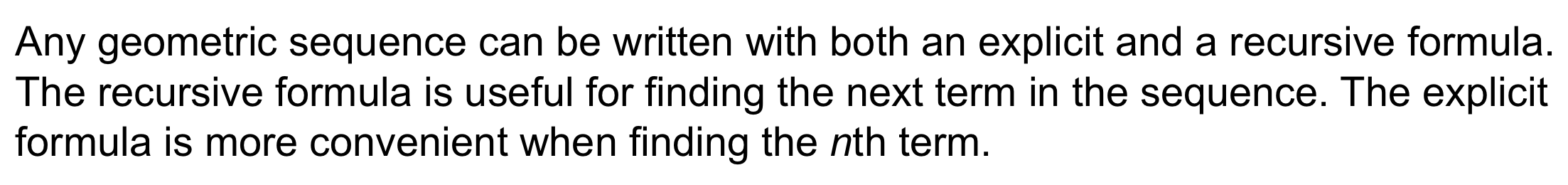6
6
10
Problem 2 Got It?
A
B
C
D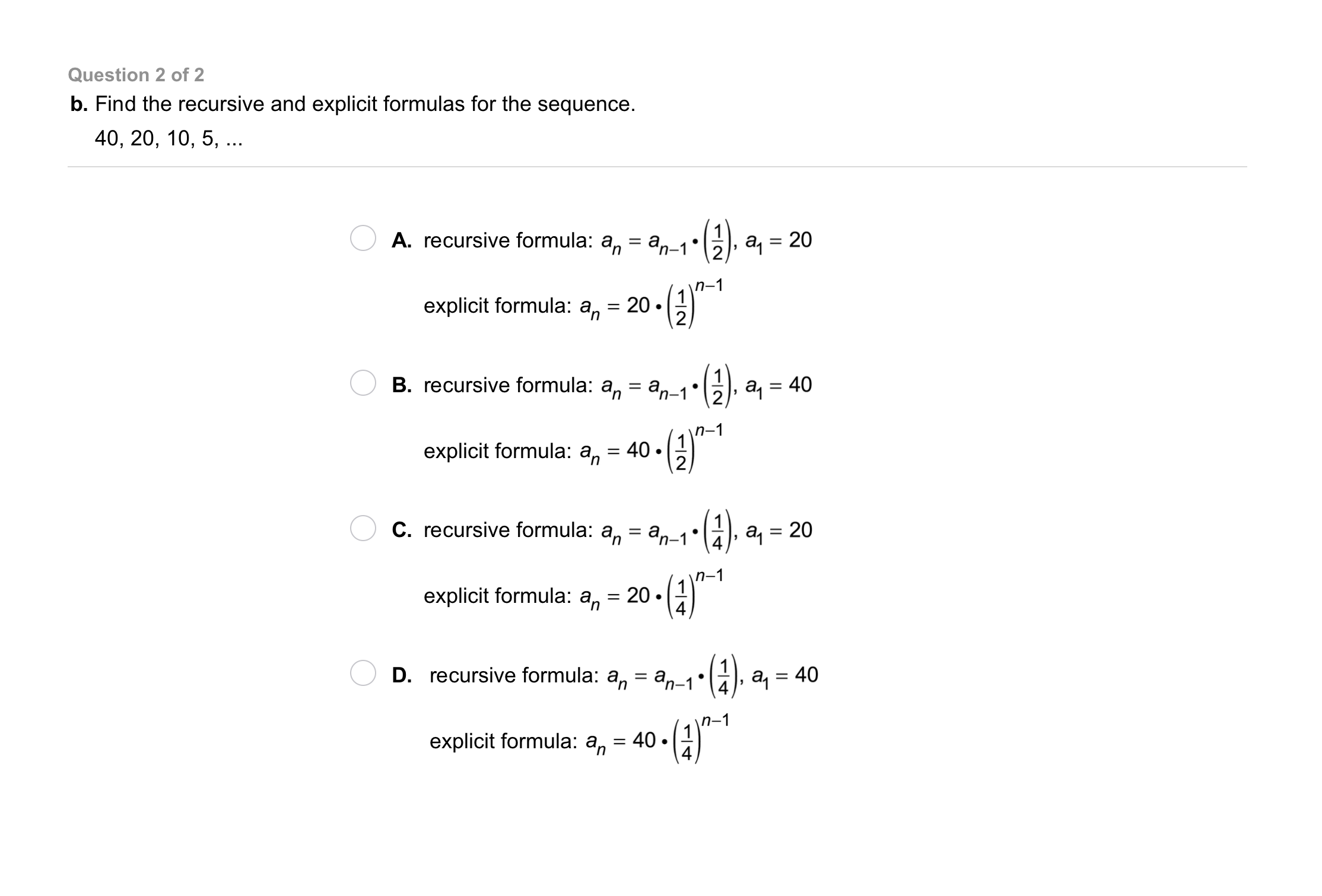7
7
10
Problem 2 Got It?
A
B
C
D
8
10
Problem 3 Got It? Write a recursive formula and an explicit formula for the sequence. Find the 8th term of the sequence.

9
10
Problem 3 Got It? Write a recursive formula and an explicit formula for the sequence. Find the 8th term of the sequence.10
20
Problem 4 Got It? A geometric sequence has an initial value of 2 and a common ratio of 3. Write a function to represent the sequence. Graph the function. Include all relevant graph detail.